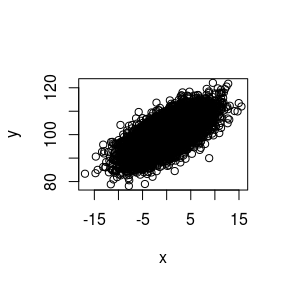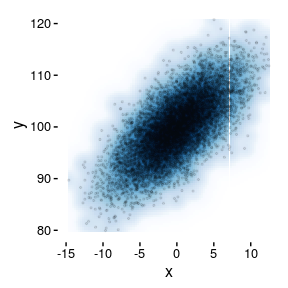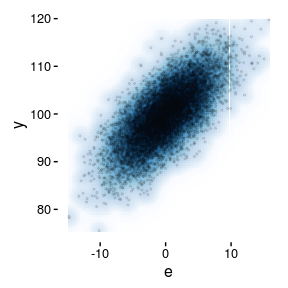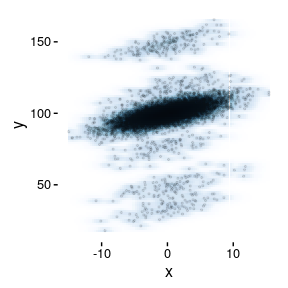saeSim provides tools for simulation studies in the context of small area estimation. It can be useful for simulation studies in the field of statistics in general if you do not get scared away by the domain specific jargon.

## An initial Example

Consider a linear mixed model. It contains two components. A fixed effects part, and an error component. The error component can be split into a random effects part and a model error.

library(saeSim)
setup <- sim_base() %>%
sim_gen_x() %>%
sim_gen_e() %>%
sim_gen_v() %>%
sim_resp_eq(y = 100 + 2 * x + v + e) %>%
sim_simName("Doku")
setup
## data.frame [10,000 x 6]
##
##      idD   idU         x          e         v         y
##    (int) (int)     (dbl)      (dbl)     (dbl)     (dbl)
## 1      1     1 -1.304933 -4.8058407 0.1329492  92.71724
## 2      1     2  5.319197 -1.9841702 0.1329492 108.78717
## 3      1     3  5.089717  0.2677245 0.1329492 110.58011
## 4      1     4  1.658566 -0.2277966 0.1329492 103.22228
## 5      1     5 -6.159800 -2.0376785 0.1329492  85.77567
## 6      1     6 -3.714268  1.5903638 0.1329492  94.29478
## ..   ...   ...       ...        ...       ...       ...

sim_base() is responsible to supply a data.frame to which variables can be added. The default is to create a data.frame with indicator variables idD and idU (2-level-model), which uniquely identify observations. ‘D’ stands for the domain, i.e. the grouping variable. ‘U’ stands for unit and is the identifier of single observations within domains. sim_resp will add a variable y as response.

The setup itself does not contain the simulated data but the functions to process the data. To start a simulation use the function sim. It will return a list containing data.frames as elements:

dataList <- sim(setup, R = 10)

You can coerce a simulation setup to a data.frame with as.data.frame.

simData <- sim_base() %>%
sim_gen_x() %>%
sim_gen_e() %>%
as.data.frame
simData

## Naming and structure

Components in a simulation setup should be added using the pipe operator %>% from magrittr. A component defines ‘when’ a specific function will be applied in a chain of functions. To add a component you can use one of: sim_gen, sim_resp, sim_comp_pop, sim_sample, sim_comp_sample, sim_agg and sim_comp_agg. They all expect a simulation setup as first argument and a function as second and will take care of the order in which the functions are called. The reason for this is the following:

setup <- sim_base() %>%
sim_gen_x() %>%
sim_gen_e() %>%
sim_resp_eq(y = 100 + 2 * x + e)

setup1 <- setup %>% sim_sample(sample_fraction(0.05))
setup2 <- setup %>% sim_sample(sample_number(5))

You can define a rudimentary scenario and only have to explain how scenarios differ. You do not have to redefine them. setup1 and setup2 only differ in the way samples are drawn. sim_sample will take care, that the sampling will take place at the appropriate place in the chain of functions no matter how setup was composed.

If you can’t remember all function names, use auto-complete. All functions to add components start with sim_. And all functions meant to be used in a given phase will start with the corresponding prefix, i.e. if you set the sampling scheme you use sim_sample – all functions to control sampling have the prefix sample.

## Exploring the setup

You will want to check your results regularly when working with sim_setup objects. Some methods are supplied to do that:

• show - this is the print method for S4-Classes. You don’t have to call show explicitly. You may have noticed that only a few lines of data are printed to the console if you evaluate a simulation setup. show will print the head of the resulting data of one simulation run.
• plot - for visualizing the data
• autoplot - Will imitate smoothScatter with ggplot2
setup <- sim_base_lmm()
plot(setup)autoplot(setup)autoplot(setup, "e")autoplot(setup %>% sim_gen_vc())## sim_gen

### Semi-custom data

saeSim has an interface to standard random number generators in R. If things get more complex you can always define new generator functions.

base_id(2, 3) %>%
sim_gen(gen_generic(rnorm, mean = 5, sd = 10, name = "x", groupVars = "idD"))
## data.frame [6 x 3]
##
##     idD   idU         x
##   (int) (int)     (dbl)
## 1     1     1 19.810178
## 2     1     2 19.810178
## 3     1     3 19.810178
## 4     2     1  2.538054
## 5     2     2  2.538054
## 6     2     3  2.538054

You can supply any random number generator to gen_generic and since we are in small area estimation you have an optional group variable to generate ‘area-level’ variables. Some short cuts for data generation are sim_gen_x, sim_gen_v and sim_gen_e which add normally distributed variables named ‘x’, ‘e’ and ‘v’ respectively. Also there are some function with the prefix ‘gen’ which will be extended in the future.

library(saeSim)
setup <- sim_base() %>%
sim_gen_x() %>% # Variable 'x'
sim_gen_e() %>% # Variable 'e'
sim_gen_v() %>% # Variable 'v' as a random-effect
sim_gen(gen_v_sar(name = "vSp")) %>% # random-effect following a SAR(1)
sim_resp_eq(y = 100 + x + v + vSp + e) # Computing 'y'
setup
## data.frame [10,000 x 7]
##
##      idD   idU         x           e         v      vSp         y
##    (int) (int)     (dbl)       (dbl)     (dbl)    (dbl)     (dbl)
## 1      1     1 -5.564211  0.90458713 -1.003117 1.429308  95.76657
## 2      1     2  3.137569  0.04030031 -1.003117 1.429308 103.60406
## 3      1     3  1.647805  1.39739005 -1.003117 1.429308 103.47139
## 4      1     4  2.611742  4.79214450 -1.003117 1.429308 107.83008
## 5      1     5  4.730168 -2.03794257 -1.003117 1.429308 103.11842
## 6      1     6 -1.778071 -6.41855638 -1.003117 1.429308  92.22956
## ..   ...   ...       ...         ...       ...      ...       ...

### Contaminated data

For contaminated data you can use the same generator functions, however, instead of using sim_gen you use sim_gen_cont which will have some extra arguments to control the contamination. To extend the above setup with a contaminated spatially correlated error component you can add the following:

contSetup <- setup %>%
sim_gen_cont(
gen_v_sar(sd = 40, name = "vSp"), # defining the model
nCont = 0.05, # 5 per cent outliers
type = "area", # whole areas are outliers, i.e. all obs within
areaVar = "idD", # var name to identify domain
fixed = TRUE # if in each iteration the same area is an outlier
)

Note that the generator is the same but with a higher standard deviation. The argument nCont controls how much observations are contaminated. Values < 1 are interpreted as probability. A single number as the number of contaminated units (can be areas or observations in each area or observations). When given with length(nCont) > 1 it will be interpreted as number of contaminated observations in each area. Use the following example to see how these things play together:

base_id(3, 4) %>%
sim_gen_x() %>%
sim_gen_e() %>%
sim_gen_ec(mean = 0, sd = 150, name = "eCont", nCont = c(1, 2, 3)) %>%
as.data.frame
##    idD idU           x           e       eCont   idC
## 1    1   1 -5.76936394  1.57875195    0.000000 FALSE
## 2    1   2  5.10966954 -1.13575855    0.000000 FALSE
## 3    1   3  0.04829011  0.72720413    0.000000 FALSE
## 4    1   4 -2.08122444 -4.09008270  121.918259  TRUE
## 5    2   1  4.27926684 -7.56686078    0.000000 FALSE
## 6    2   2 -4.35999044 -1.42744326    0.000000 FALSE
## 7    2   3 -5.66198947 -5.27208606 -171.042149  TRUE
## 8    2   4  3.88111082 -2.31423540   10.860654  TRUE
## 9    3   1  0.71092894 -7.74332696    0.000000 FALSE
## 10   3   2  0.41087257  0.07353711 -152.712028  TRUE
## 11   3   3 -2.04870396  0.75518776  117.275199  TRUE
## 12   3   4 -7.70567255 -1.55844756    1.653595  TRUE

## sim_comp

Here follow some examples how to add components for computation to a sim_setup. Three points can be accessed with

• sim_comp_pop - add a computation before sampling
• sim_comp_sample - add a computation after sampling
• sim_comp_agg - add a computation after aggregation
base_id(2, 3) %>%
sim_gen_x() %>%
sim_gen_e() %>%
sim_gen_ec() %>%
sim_resp_eq(y = 100 + x + e) %>%
# the mean in each domain:
sim_comp_pop(comp_var(popMean = mean(y)), by = "idD")
## data.frame [6 x 7]
##
##     idD   idU          x           e   idC         y   popMean
##   (int) (int)      (dbl)       (dbl) (lgl)     (dbl)     (dbl)
## 1     1     1 -0.7130757 -1.14531664 FALSE  98.14161 105.14508
## 2     1     2  6.1185331  1.52568769 FALSE 107.64422 105.14508
## 3     1     3  3.9817541  5.66766927 FALSE 109.64942 105.14508
## 4     2     1  0.5455610  0.07964931 FALSE 100.62521  97.57322
## 5     2     2 -5.8344649 -0.48614937 FALSE  93.67939  97.57322
## 6     2     3  4.1438907 -5.72882258 FALSE  98.41507  97.57322

The function comp_var is a wrapper around dplyr::mutate so you can add simple data manipulations. The argument by is a little extension and lets you define operations in the scope of groups identified by a variable in the data. In this case the mean of the variable ‘y’ is computed for every group identified with the variable ‘idD’. This is done before sampling, hence the prefix ‘pop’ for population.

By adding computation functions you can extend the functionality to wrap your whole simulation. This will separate the utility of this package from only generating data.

comp_linearPredictor <- function(dat) {
dat\$linearPredictor <- lm(y ~ x, dat) %>% predict
dat
}

sim_base_lm() %>%
sim_comp_pop(comp_linearPredictor)
## data.frame [10,000 x 6]
##
##      idD   idU          x          e         y linearPredictor
##    (int) (int)      (dbl)      (dbl)     (dbl)           (dbl)
## 1      1     1  0.7814646 -6.9970485  93.78442       100.81580
## 2      1     2  3.2184158  1.5238232 104.74224       103.28009
## 3      1     3 -1.6970104  0.4080286  98.71102        98.30952
## 4      1     4  1.3630016 -1.0567830 100.30622       101.40386
## 5      1     5 -3.6160438 -4.3465426  92.03741        96.36896
## 6      1     6  3.0740573 -0.8895065 102.18455       103.13411
## ..   ...   ...        ...        ...       ...             ...

Or, should this be desirable, directly produce a list of lm objects or add them as attribute to the data. The intended way of writing functions is that they will return the modified data of class ‘data.frame’.

sim_base_lm() %>%
sim_comp_pop(function(dat) lm(y ~ x, dat)) %>%
sim(R = 1)
## []
##
## Call:
## lm(formula = y ~ x, data = dat)
##
## Coefficients:
## (Intercept)            x
##     99.9970       0.9936
comp_linearModelAsAttr <- function(dat) {
attr(dat, "linearModel") <- lm(y ~ x, dat)
dat
}

dat <- sim_base_lm() %>%
sim_comp_pop(comp_linearModelAsAttr) %>%
as.data.frame

attr(dat, "linearModel")
##
## Call:
## lm(formula = y ~ x, data = dat)
##
## Coefficients:
## (Intercept)            x
##     100.040        1.007

If you use any kind of sampling, the ‘linearPredictor’ can be added after sampling. This is where small area models are supposed to be applied.

sim_base_lm() %>%
sim_sample() %>%
sim_comp_sample(comp_linearPredictor)
## data.frame [500 x 6]
##
##      idD   idU         x         e         y linearPredictor
##    (int) (int)     (dbl)     (dbl)     (dbl)           (dbl)
## 1      1    53 -2.647857 -5.584788  91.76736        97.10895
## 2      1    13 -4.644544 -4.702967  90.65249        95.13370
## 3      1    28  5.700598 -6.211777  99.48882       105.36774
## 4      1    49 -1.453102 -4.277282  94.26962        98.29087
## 5      1    59  2.229623 -1.741713 100.48791       101.93404
## 6      2     8 -1.373660  4.732568 103.35891        98.36946
## ..   ...   ...       ...       ...       ...             ...

Should you want to apply area level models, use sim_comp_agg instead.

sim_base_lm() %>%
sim_sample() %>%
sim_agg() %>%
sim_comp_agg(comp_linearPredictor)
## data.frame [100 x 5]
##
##      idD         x           e         y linearPredictor
##    (dbl)     (dbl)       (dbl)     (dbl)           (dbl)
## 1      1 2.0803441  0.29126868 102.37161        102.4466
## 2      2 0.5225518 -2.93530963  97.58724        100.6507
## 3      3 0.1169371 -3.38964340  96.72729        100.1831
## 4      4 2.3195498  2.20774517 104.52729        102.7224
## 5      5 0.9490940  0.03359516 100.98269        101.1424
## 6      6 2.0931435  1.31084935 103.40399        102.4613
## ..   ...       ...         ...       ...             ...

## sim_sample

After the data generation you may want to draw a sample from the population. Use the function sim_sample() to add a sampling component to your sim_setup.

• sample_number - wrapper around dplyr::sample_n
• sample_fraction - wrapper around dplyr::sample_frac
base_id(3, 4) %>%
sim_gen_x() %>%
sim_sample(sample_number(1L))
## data.frame [1 x 3]
##
##     idD   idU         x
##   (int) (int)     (dbl)
## 1     2     3 0.1723437
base_id(3, 4) %>%
sim_gen_x() %>%
sim_sample(sample_number(1L, groupVars = "idD"))
## data.frame [3 x 3]
##
##     idD   idU          x
##   (int) (int)      (dbl)
## 1     1     1  5.6768604
## 2     2     4 -1.3498366
## 3     3     2  0.1335789
# simple random sampling:
sim_base_lm() %>% sim_sample(sample_number(size = 10L))
## data.frame [10 x 5]
##
##      idD   idU          x          e         y
##    (int) (int)      (dbl)      (dbl)     (dbl)
## 1     42     1  5.3957874  0.5252143 105.92100
## 2     67    71 -2.6640517  4.6646576 102.00061
## 3     76    97 -7.7902988  0.5985310  92.80823
## 4     92    36 -0.8942010 -1.7083084  97.39749
## 5     50    43  2.2193835 -3.2735544  98.94583
## 6     57    34  0.4940237  5.2446039 105.73863
## ..   ...   ...        ...        ...       ...
sim_base_lm() %>% sim_sample(sample_fraction(size = 0.05))
## data.frame [500 x 5]
##
##      idD   idU         x         e         y
##    (int) (int)     (dbl)     (dbl)     (dbl)
## 1     98     2  2.168069 -2.451258  99.71681
## 2     86    68 -1.726156 -4.423079  93.85077
## 3     33    11 -2.415454  3.918225 101.50277
## 4      4    74  4.371700 -4.960200  99.41150
## 5      3    69 -3.418516 -2.071981  94.50950
## 6     26    59  1.595793 -3.244890  98.35090
## ..   ...   ...       ...       ...       ...
# srs in each domain/cluster
sim_base_lm() %>% sim_sample(sample_number(size = 10L, groupVars = "idD"))
## data.frame [1,000 x 5]
##
##      idD   idU          x          e         y
##    (int) (int)      (dbl)      (dbl)     (dbl)
## 1      1    25  2.8688381 -0.1487975 102.72004
## 2      1    50  0.4623502  2.7232846 103.18563
## 3      1    79 -4.7694712  1.4317169  96.66225
## 4      1    31  3.0863110  1.1893247 104.27564
## 5      1    12  4.2486439  1.5025416 105.75119
## 6      1    93  6.9106009 -4.1697016 102.74090
## ..   ...   ...        ...        ...       ...
sim_base_lm() %>% sim_sample(sample_fraction(size = 0.05, groupVars = "idD"))
## data.frame [500 x 5]
##
##      idD   idU           x          e         y
##    (int) (int)       (dbl)      (dbl)     (dbl)
## 1      1    61  4.44641857 -3.9413459 100.50507
## 2      1    33  0.05282711  1.6759231 101.72875
## 3      1    20 -7.54034854  2.2487514  94.70840
## 4      1    41 -3.95023671  0.7891577  96.83892
## 5      1    99  1.44413010  0.4178455 101.86198
## 6      2    28  9.09919382 -0.4214601 108.67773
## ..   ...   ...         ...        ...       ...